Plot a population pyramid (age-sex) from a dataframe.

age_pyramid(
data,
age_group = "age_group",
split_by = "sex",
stack_by = NULL,
count = NULL,
proportional = FALSE,
na.rm = TRUE,
show_midpoint = TRUE,
vertical_lines = FALSE,
horizontal_lines = TRUE,
pyramid = TRUE,
pal = NULL
)

## Arguments

data Your dataframe (e.g. linelist) the name of a column in the data frame that defines the age group categories. Defaults to "age_group" the name of a column in the data frame that defines the the bivariate column. Defaults to "sex". See NOTE the name of the column in the data frame to use for shading the bars. Defaults to NULL which will shade the bars by the split_by variable. for pre-computed data the name of the column in the data frame for the values of the bars. If this represents proportions, the values should be within [0, 1]. If TRUE, bars will represent proportions of cases out of the entire population. Otherwise (FALSE, default), bars represent case counts If TRUE, this removes NA counts from the age groups. Defaults to TRUE. When TRUE (default), a dashed vertical line will be added to each of the age bars showing the halfway point for the un-stratified age group. When FALSE, no halfway point is marked. If you would like to add dashed vertical lines to help visual interpretation of numbers. Default is to not show (FALSE), to turn on write TRUE. If TRUE (default), horizontal dashed lines will appear behind the bars of the pyramid if TRUE, then binary split_by variables will result in a population pyramid (non-binary variables cannot form a pyramid). If FALSE, a pyramid will not form. a color palette function or vector of colors to be passed to ggplot2::scale_fill_manual() defaults to the first "qual" palette from ggplot2::scale_fill_brewer().

## Note

If the split_by variable is bivariate (e.g. an indicator for a specific symptom), then the result will show up as a pyramid, otherwise, it will be presented as a facetted barplot with with empty bars in the background indicating the range of the un-facetted data set. Values of split_by will show up as labels at top of each facet.

## Examples


library(ggplot2)
old <- theme_set(theme_classic(base_size = 18))

# with pre-computed data ----------------------------------------------------
# 2018/2008 US census data by age and gender
data(us_2018)
data(us_2008)
age_pyramid(us_2018, age_group = age, split_by = gender, count = count)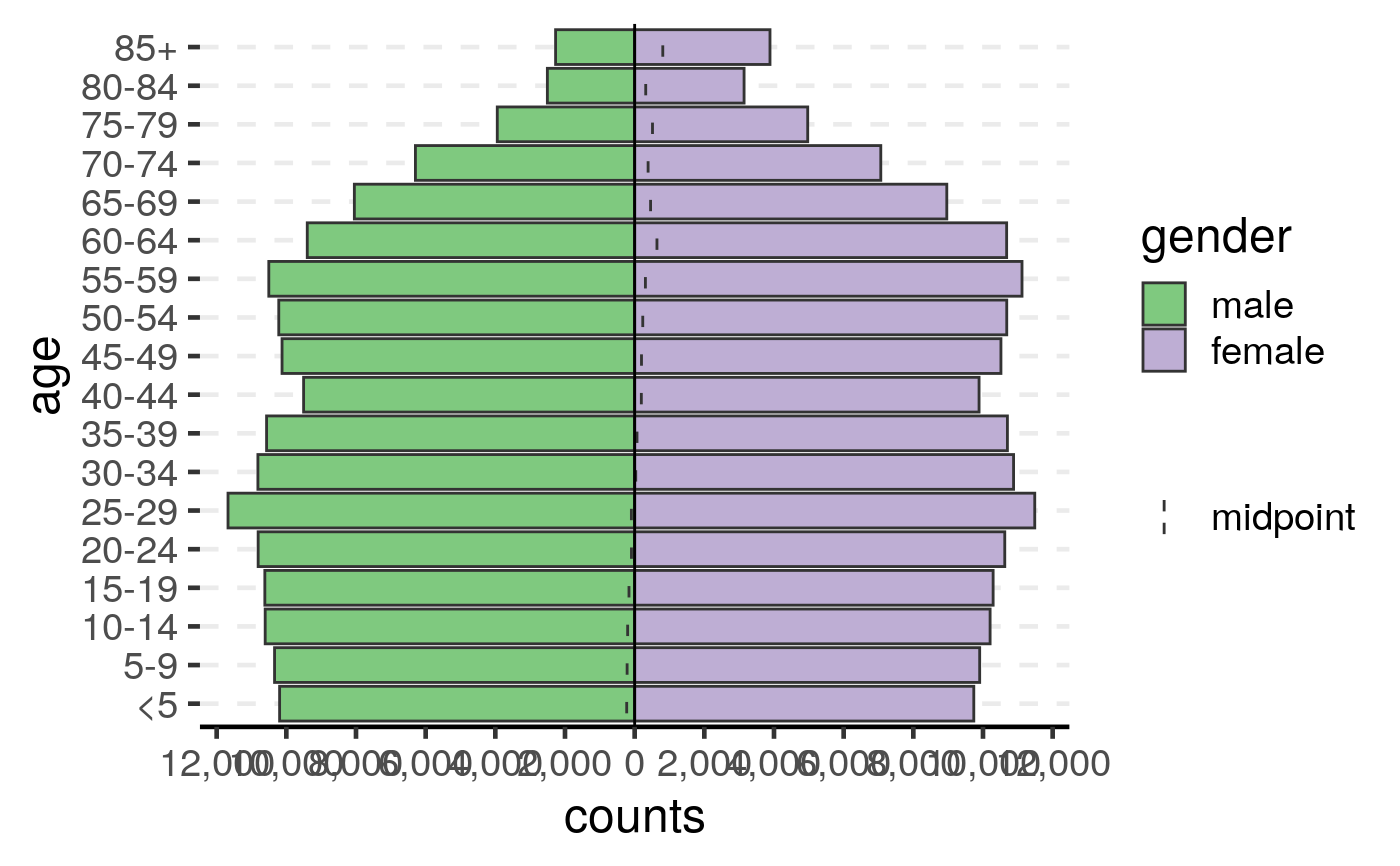age_pyramid(us_2008, age_group = age, split_by = gender, count = count)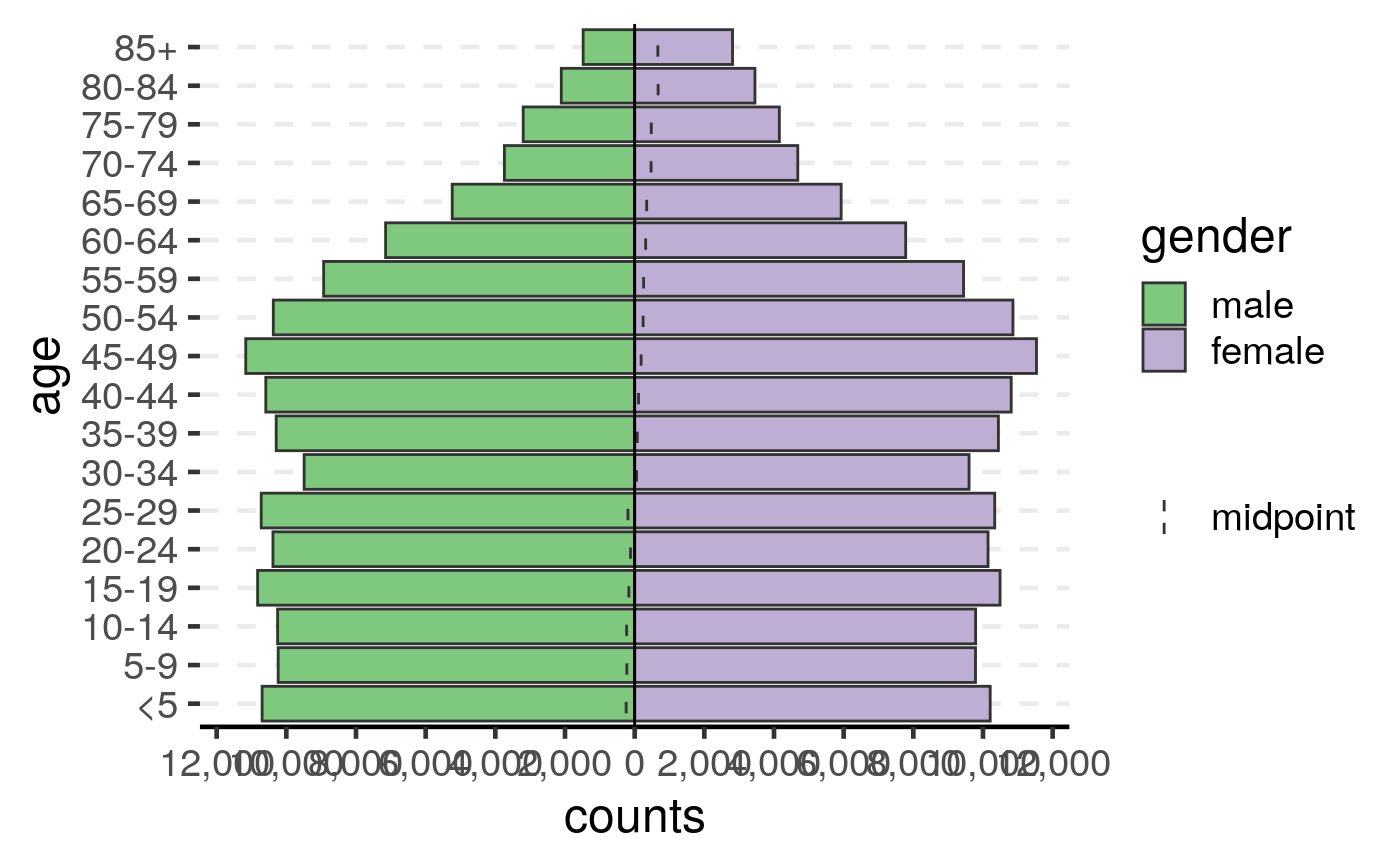# 2018 US census data by age, gender, and insurance status
data(us_ins_2018)
age_pyramid(us_ins_2018,
age_group = age,
split_by = gender,
stack_by = insured,
count = count
)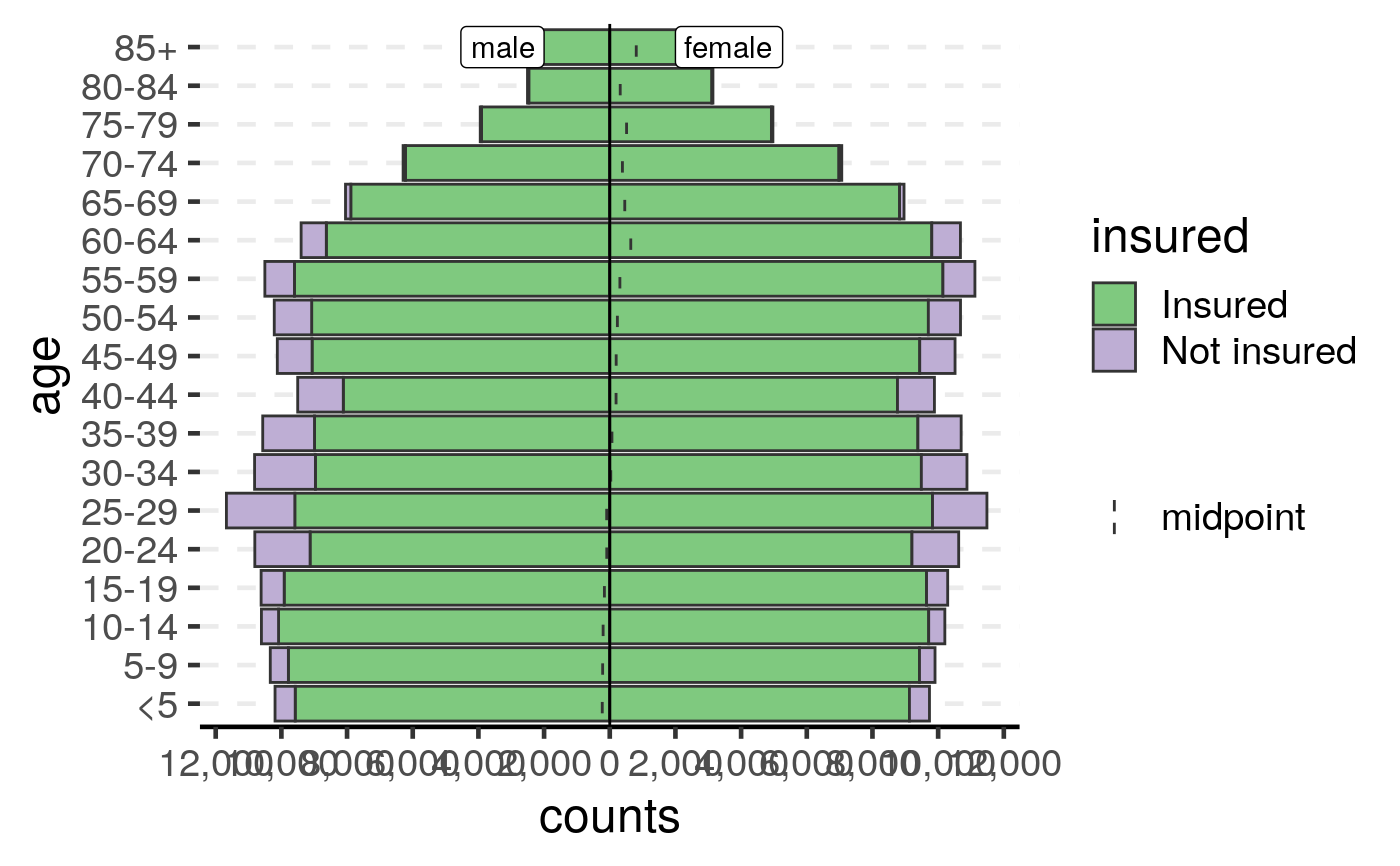us_ins_2018$prop <- us_ins_2018$percent/100
age_pyramid(us_ins_2018,
age_group = age,
split_by = gender,
stack_by = insured,
count = prop,
proportion = TRUE
)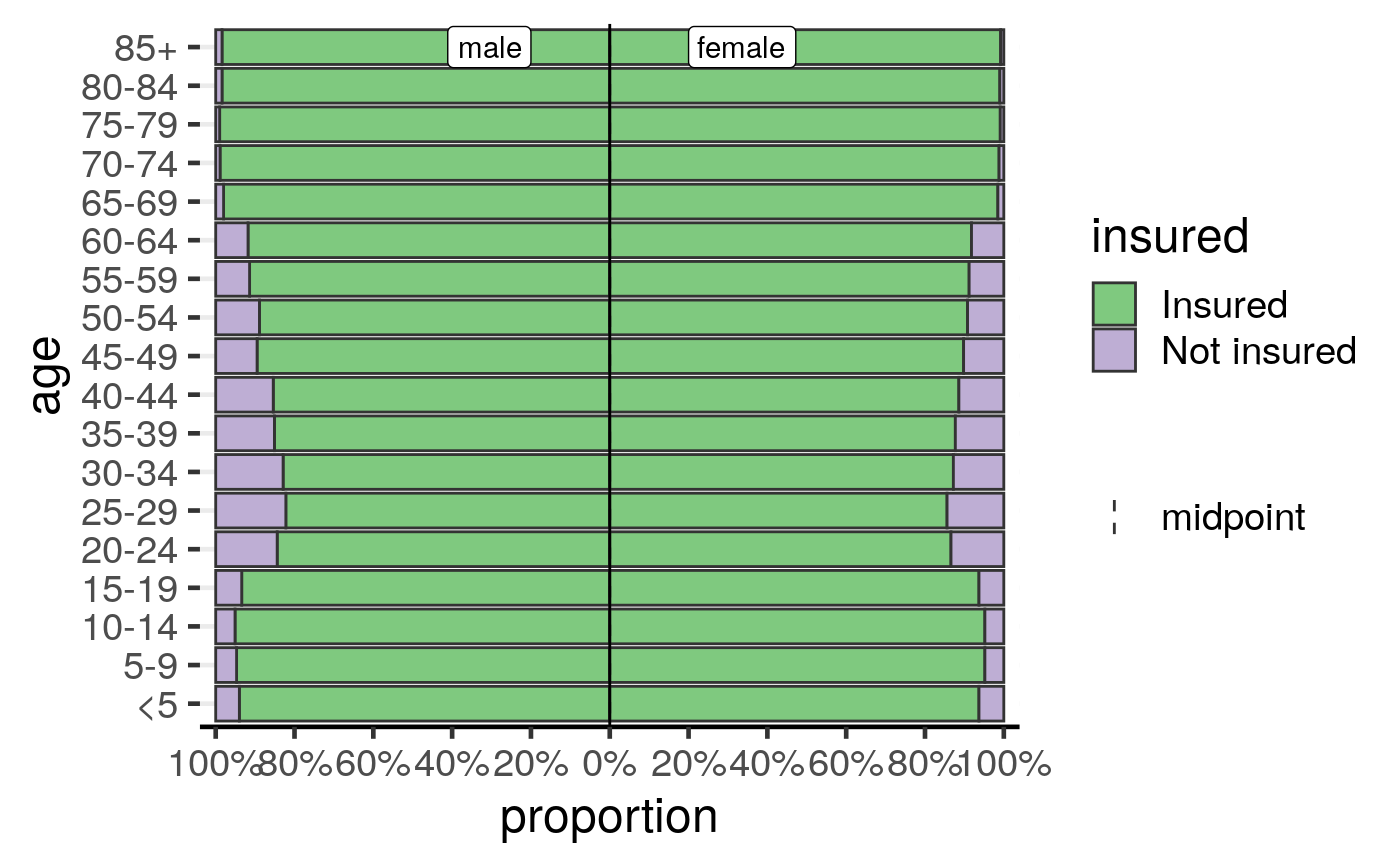# from linelist data --------------------------------------------------------
set.seed(2018 - 01 - 15)
ages <- cut(sample(80, 150, replace = TRUE),
breaks = c(0, 5, 10, 30, 90), right = FALSE
)
sex <- sample(c("Female", "Male"), 150, replace = TRUE)
gender <- sex
gender[sample(5)] <- "NB"
ill <- sample(c("case", "non-case"), 150, replace = TRUE)
dat <- data.frame(
AGE = ages,
sex = factor(sex, c("Male", "Female")),
gender = factor(gender, c("Male", "NB", "Female")),
ill = ill,
stringsAsFactors = FALSE
)

# Create the age pyramid, stratifying by sex
print(ap <- age_pyramid(dat, age_group = AGE))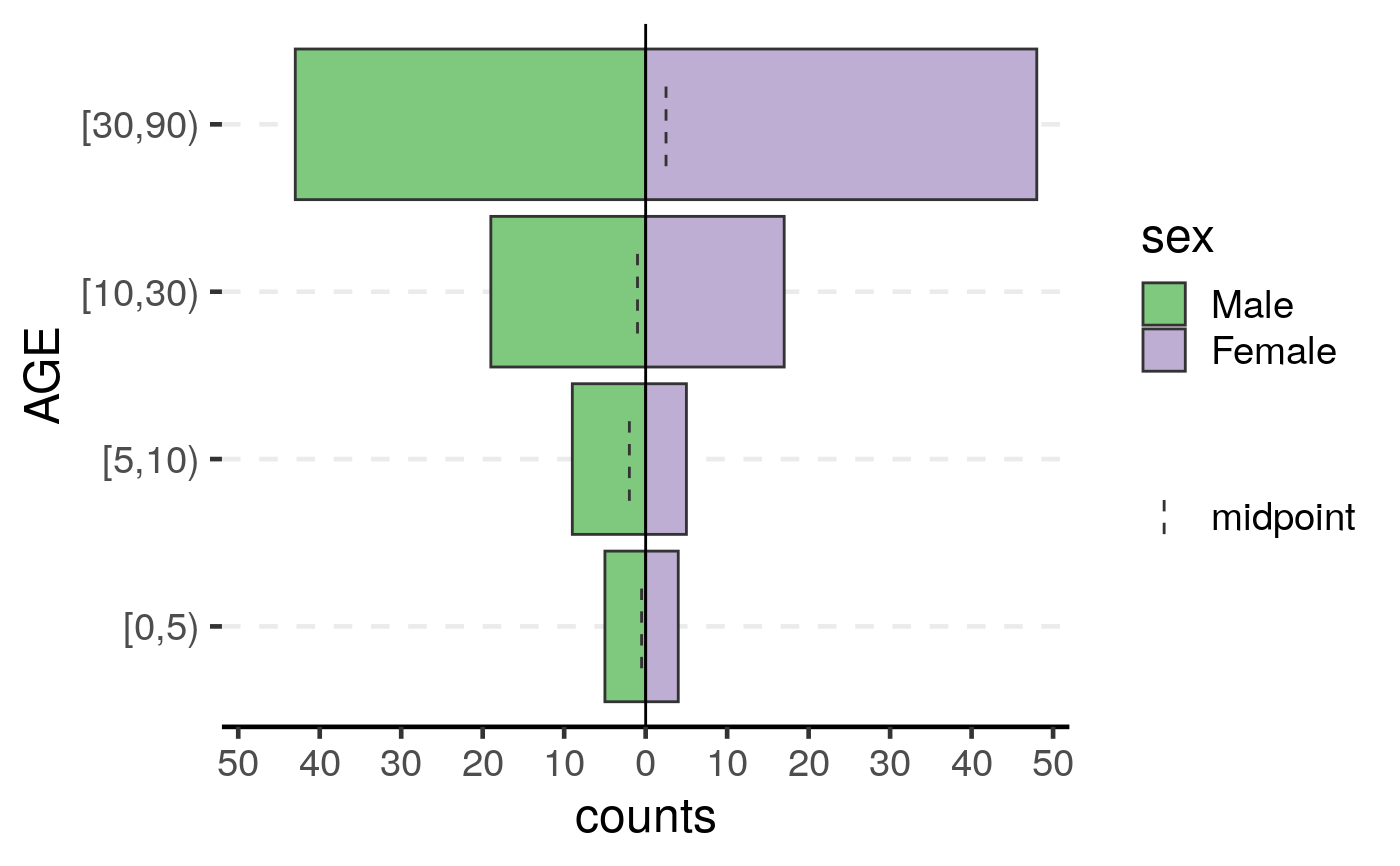# Create the age pyramid, stratifying by gender, which can include non-binary
print(apg <- age_pyramid(dat, age_group = AGE, split_by = gender))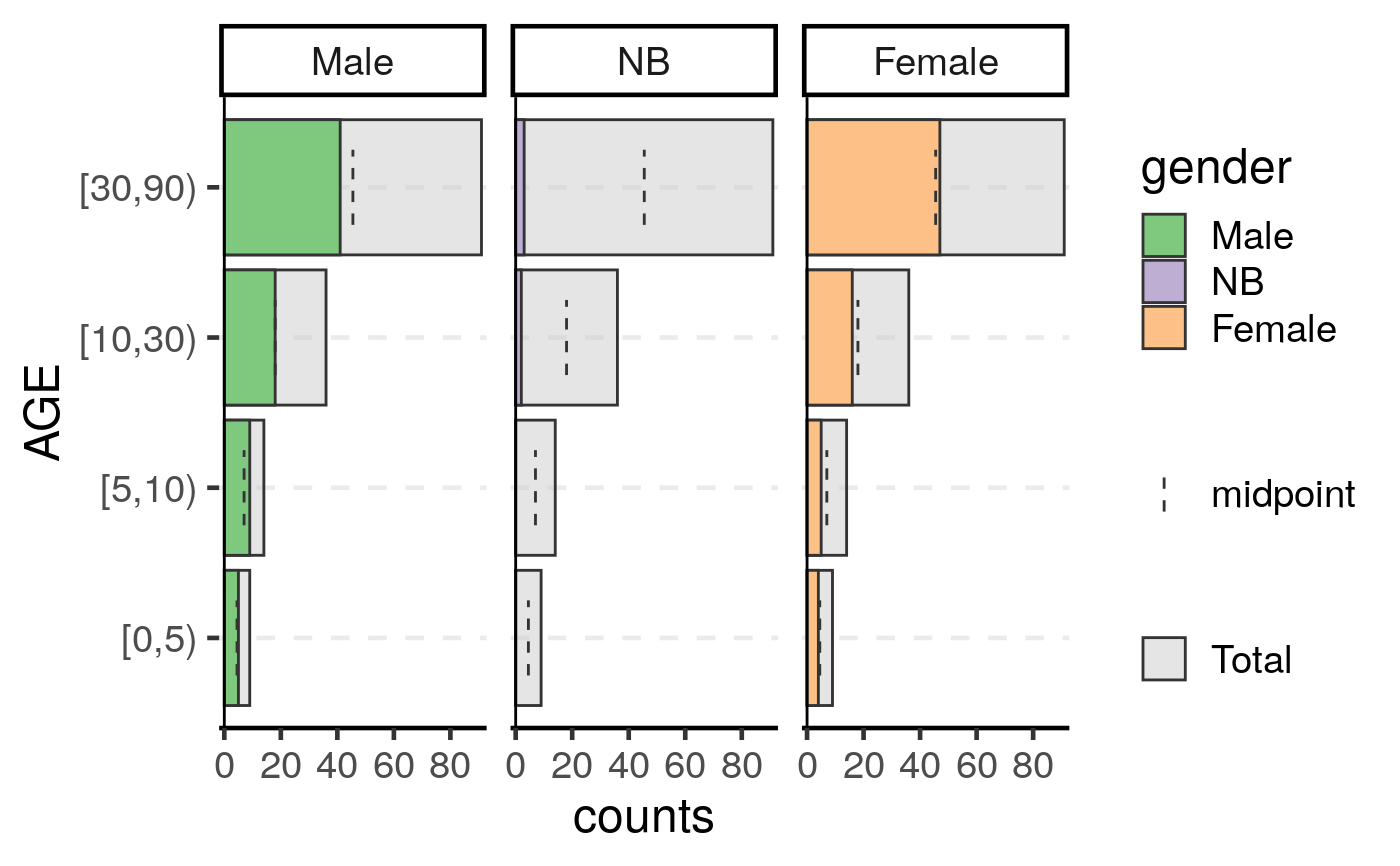# Remove NA categories with na.rm = TRUE
dat2 <- dat
dat2[1, 1] <- NA
dat2[2, 2] <- NA
dat2[3, 3] <- NA
print(ap <- age_pyramid(dat2, age_group = AGE))#> Warning: 2 missing rows were removed (1 values from AGE and 1 values from sex).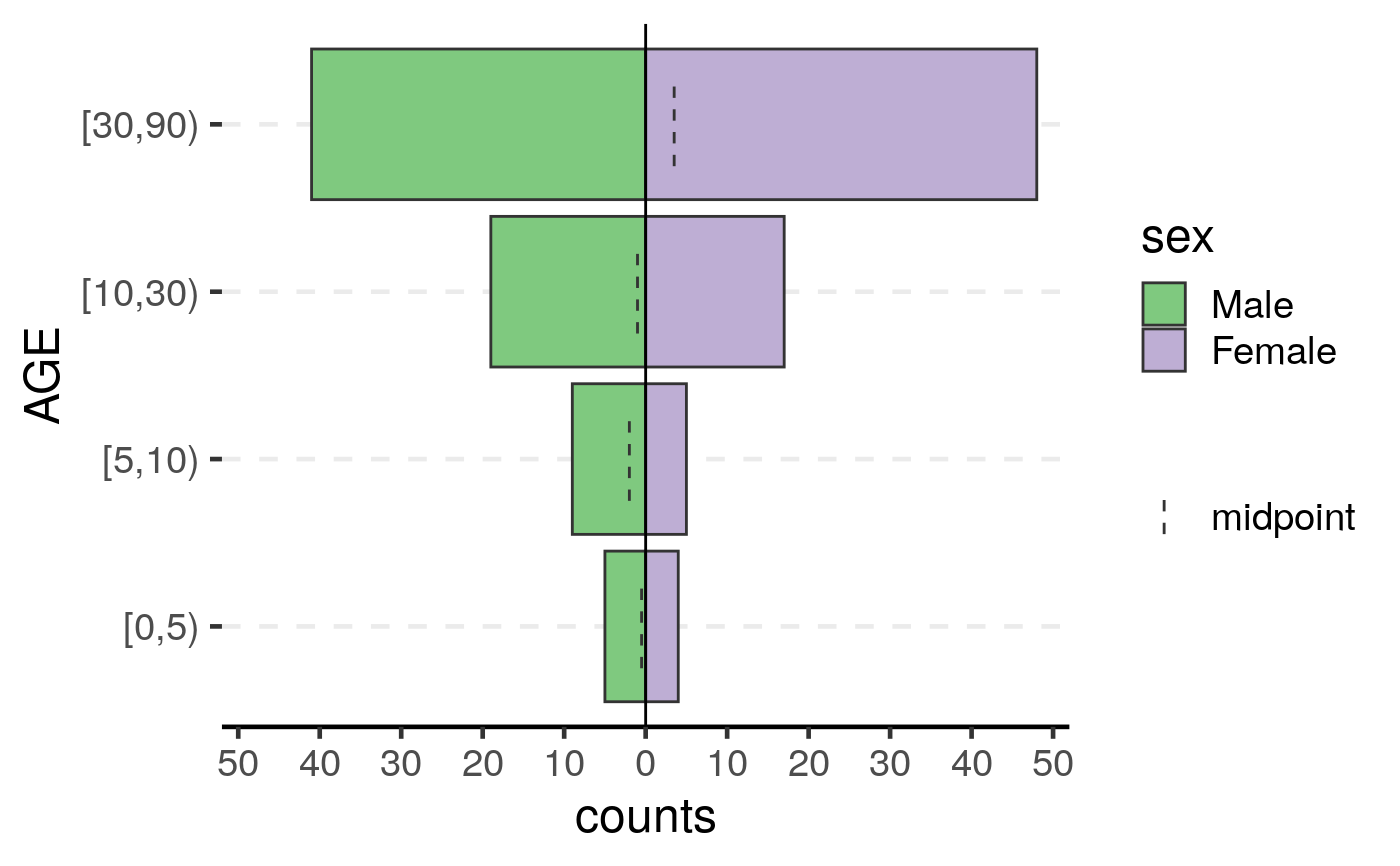print(ap <- age_pyramid(dat2, age_group = AGE, na.rm = TRUE))#> Warning: 2 missing rows were removed (1 values from AGE and 1 values from sex).# Stratify by case definition and customize with ggplot2
ap <- age_pyramid(dat, age_group = AGE, split_by = ill) +
theme_bw(base_size = 16) +
labs(title = "Age groups by case definition")
print(ap)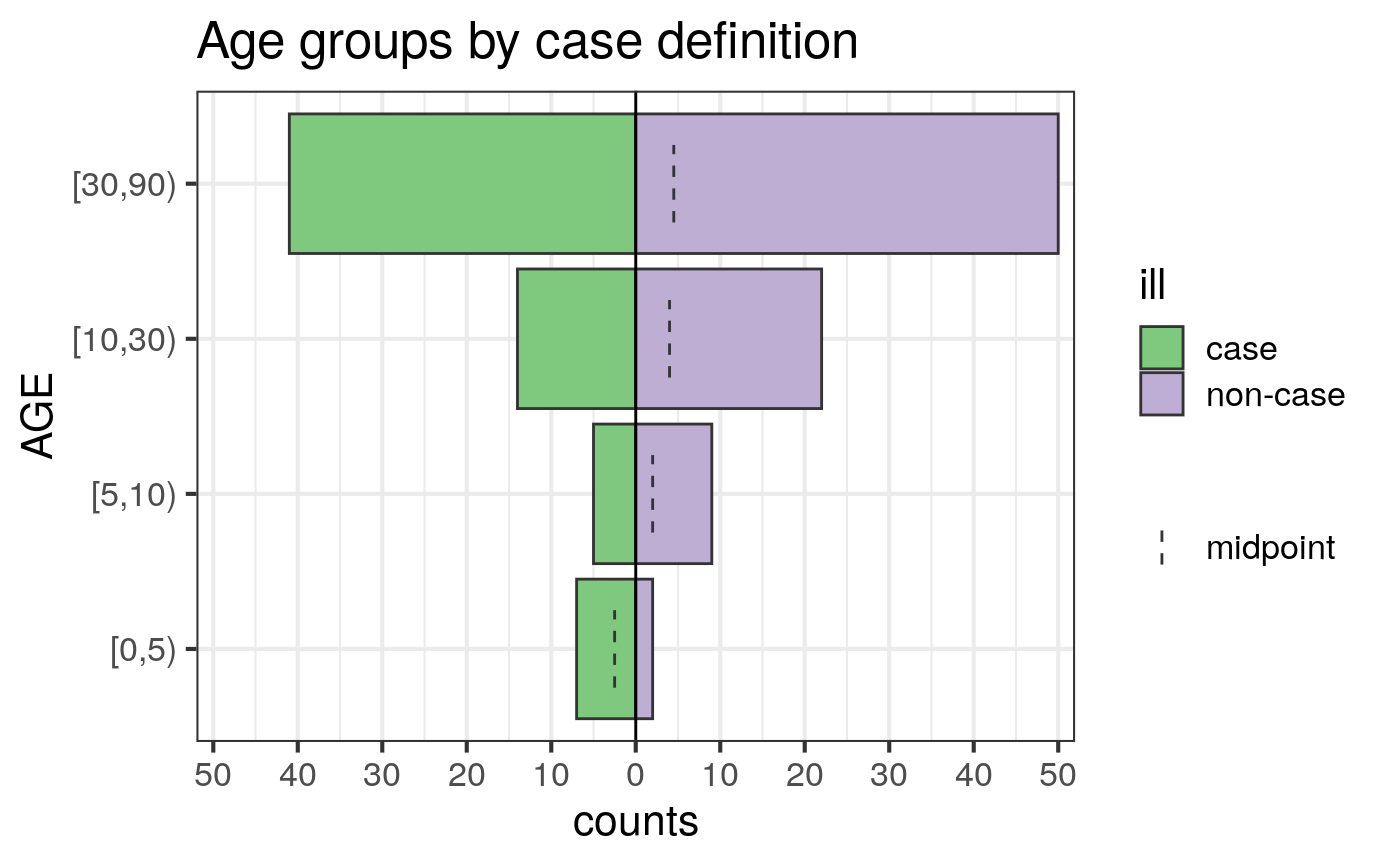# Stratify by multiple factors
ap <- age_pyramid(dat,
age_group = AGE,
split_by = sex,
stack_by = ill,
vertical_lines = TRUE
) +
labs(title = "Age groups by case definition and sex")
print(ap)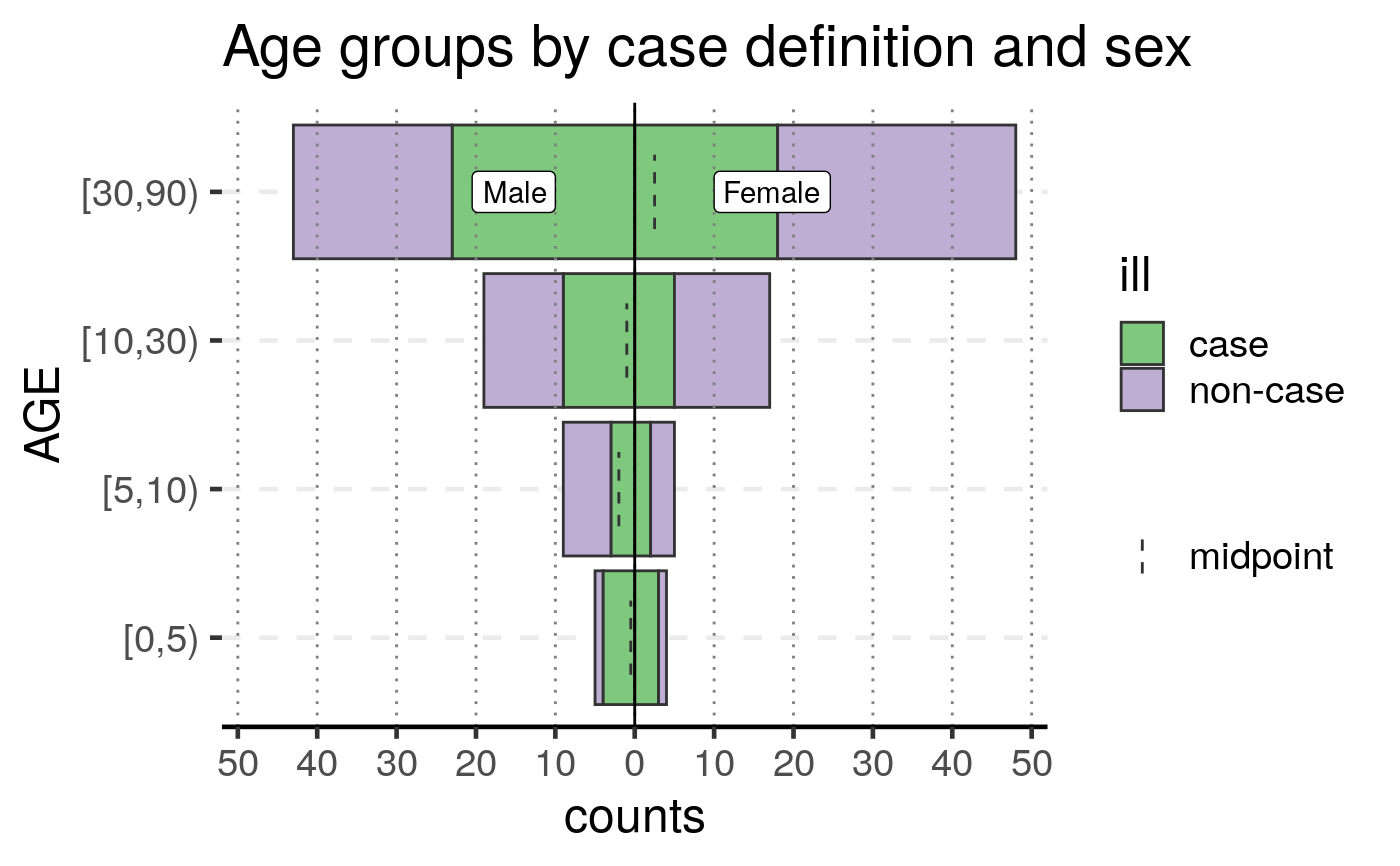# Display proportions
ap <- age_pyramid(dat,
age_group = AGE,
split_by = sex,
stack_by = ill,
proportional = TRUE,
vertical_lines = TRUE
) +
labs(title = "Age groups by case definition and sex")
print(ap)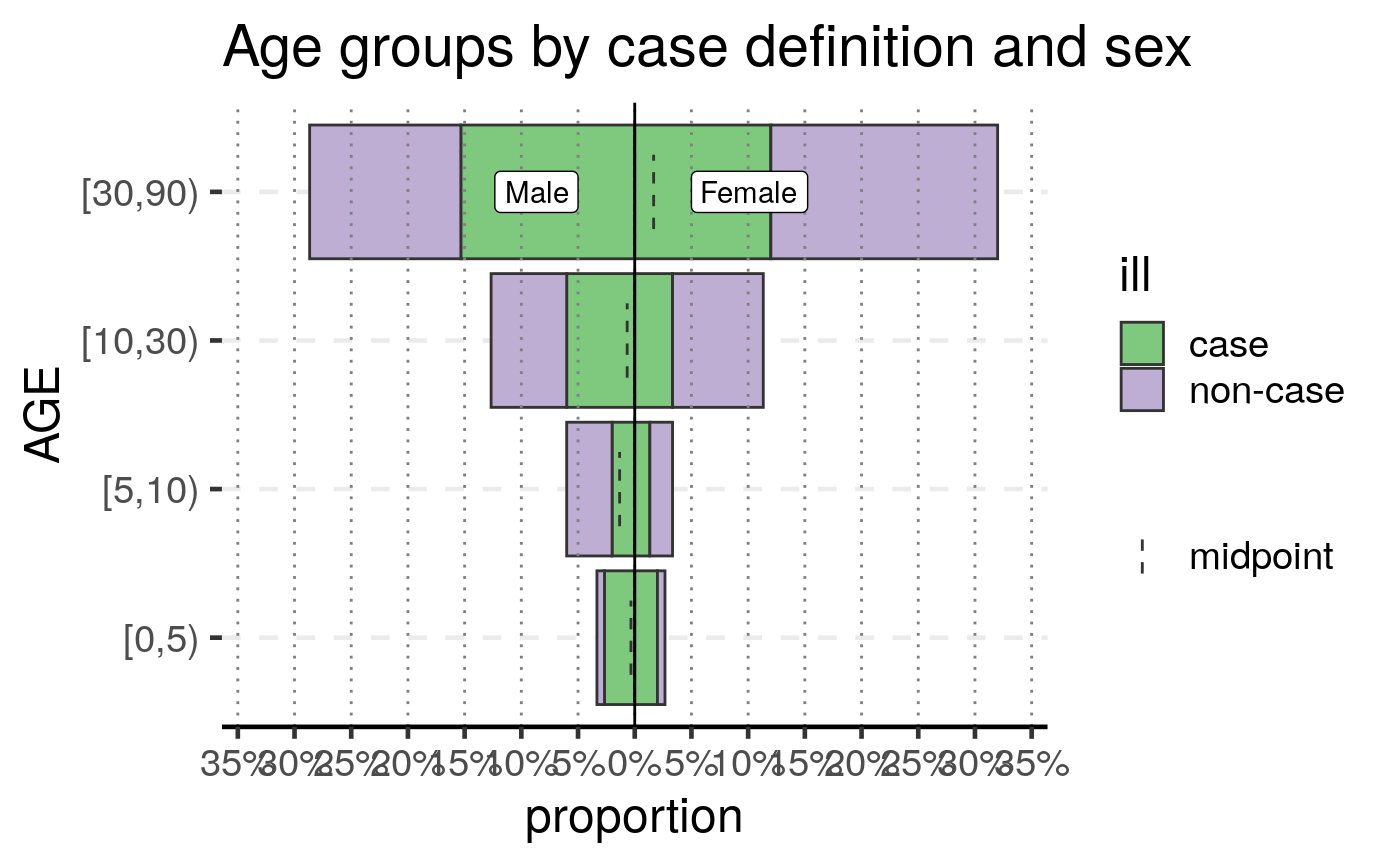# empty group levels will still be displayed
dat3 <- dat2
dat3[dat\$AGE == "[0,5)", "sex"] <- NA
age_pyramid(dat3, age_group = AGE)#> Warning: 11 missing rows were removed (1 values from AGE and 10 values from sex).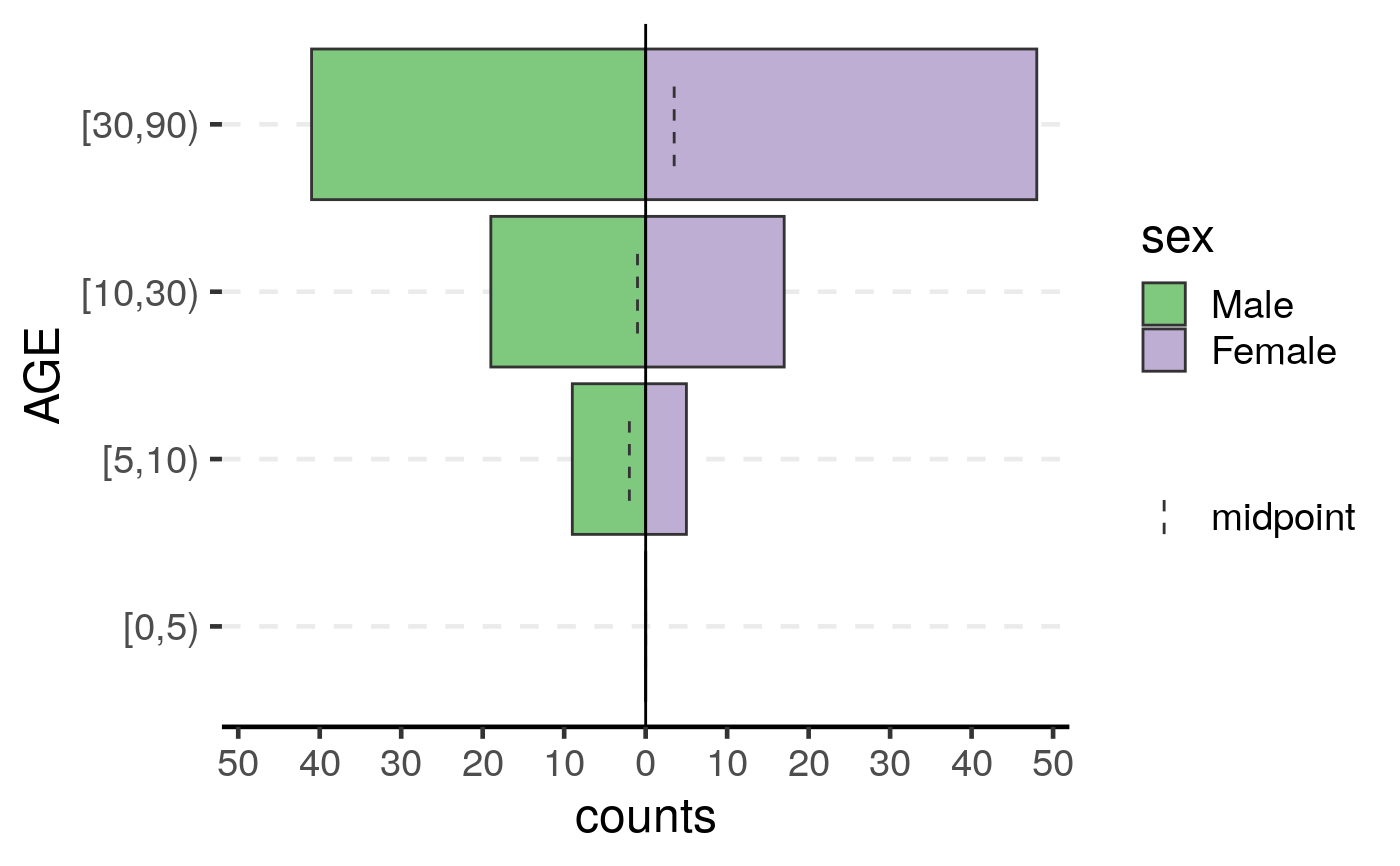theme_set(old)